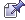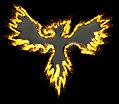###Author Topic: Vector Math Library - Vector as an object, OOP Style.  (Read 17820 times)

####Hemlos

• To boldy go where no pixel has gone before!
• Global Moderator
• Prof. Inline
••• Posts: 1622
• Particle Hawk##### Vector Math Library - Vector as an object, OOP Style.
« on: 2015-Aug-30 »
This Vector lib can be used for 2d or 3d.
The vector is treated as an object, hence OOP style.
It transforms, or analises the vectors as an object.
All known, fundamental, vector functions should be found in this lib, if there is something missing please let me know.
This lib is very stable and tested using Sir Isaac Newton's law of universal gravitation. (in my yet to be revealed game)A. ||V|| = 1 (it should anyway, if not let me know how!)
B. Can be used to set the absolute length. eg. instead of using a second SCALAR. saves some CPU steps!

VEC3:

Code: (glbasic) [Select]
`// --------------------------------- //// Project: Vector Object - OOP// Start: Saturday, August 29, 2015// Created By: Neil M. Silver// Code based on the works done by BigSofty and Jestermon.//// ======================================================================================== //// Vector Object - OOP//// This is a Vector Library, true OOP style.// The Vector object can be analised, or transformed with vector math functions.//// Note:// Declare your vectors ahead of time AS VEC3.// Your main vector is the main object(VecA), and you pass in secondary vectors(VecB) as inputs.// VecB as an input is always the OTHER secondary vector being analised.// CrossProduct function outputs VectorOutput AS Vec3 as the output, to preserve the first vector.//// LOCAL My3dVector AS VEC3// My3dVector._INIT_(1.0,1.0,1.0) //not a normalized vector.// My3dVector._NORMALIZE()  //My3dVector length is now normalized to 1 which preserves its original direction, eg ||V|| = 1// My3dVector._SCALE(2.0) // vector is now length = 2, in its original direction.//// LOCAL My3dVector2 AS VEC3// My3dVector2._INIT_(3.0,-1.0,-2.0) //not a normalized vector.// LOCAL MyProductOutputVector AS VEC3 // no need to init this one...itll be used for the product results.// My3dVector._CROSSPRODUCT(My3dVector2, MyProductOutputVector ) //MyProductOutputVector contains the results of this function byref.//TYPE VEC3 // 3 dimensional Vector Object //VecA vX# vY# vZ# // Initialise Your Vector Object Here. FUNCTION _INIT_: vX#, vY#, vZ# // LIB VEC3 - Vector Object Input self.vX# = vX# self.vY# = vY# self.vZ# = vZ# ENDFUNCTION// Information Analysis function sets: // DOT Product // Note, Computers arent reliable calcultating DOT // IF value is < 0.01 THEN it is really 0! // I tested many different values in a vector calculator to find this tolerance number. // That said, this DOT function works really well. FUNCTION _DOT#: VecB AS VEC3 // LIB VEC3 - Vector DOT Product LOCAL DOT# = ( (self.vX*VecB.vX) + (self.vY*VecB.vY) + (self.vZ*VecB.vZ) ) IF DOT < 0.01 THEN DOT = 0 RETURN DOT ENDFUNCTION // Magnitude FUNCTION _MAG#: // LIB VEC3 - Vector Magnitude RETURN SQR( (self.vX*self.vX) + (self.vY*self.vY) + (self.vZ*self.vZ) ) ENDFUNCTION // Cross Product // Returns VecOutput with a vector cross product as its value. FUNCTION _CROSS: VecB AS VEC3, VecOutput AS VEC3 // LIB VEC3 - Cross Product - VecOutput is the output.     VecOutput.vX = (self.vY * VecB.vZ) - (self.vZ * VecB.vY)     VecOutput.vY = (self.vZ * VecB.vX) - (self.vX * VecB.vZ)     VecOutput.vZ = (self.vX * VecB.vY) - (self.vY * VecB.vX) ENDFUNCTION// Vector Math Section, These Mutate/Transform/Affect your Vector Object: // Normalize, Vector Length = 1 (eg. ||V|| = 1) // You can change the Size of the normal, mutates vector absolute size as the product =D // This is the same as normalizing your vector then scaling it up! // Much faster to scale the absolute size here initially, rather than to normailize and then scale up, saves steps. // If you dont feel comfortable with this, then use the regular vector _SCALAR function after normalizing to 1. // Leave it as 1.0 for a normal normalization product. FUNCTION _NORMALIZE: Size# = 1.0  // LIB VEC3 - Normalize the Vector to length = 1.0 LOCAL vLEN# = self._MAG() self.vX# = self.vX#  * ( Size# / vLEN ) self.vY# = self.vY#  * ( Size# / vLEN ) self.vZ# = self.vZ#  * ( Size# / vLEN ) ENDFUNCTION FUNCTION _ADD: VecB AS VEC3 // LIB VEC3 - Vector Addition self.vX = self.vX + VecB.vX self.vY = self.vY + VecB.vY self.vZ = self.vZ + VecB.vZ ENDFUNCTION FUNCTION _SUBTRACT: VecB AS VEC3 // LIB VEC3 - Vector Subtraction self.vX = self.vX - VecB.vX self.vY = self.vY - VecB.vY self.vZ = self.vZ - VecB.vZ ENDFUNCTION FUNCTION _SCALAR: Scale# // LIB VEC3 - Vector Scalar self.vX = self.vX * Scale self.vY = self.vY * Scale self.vZ = self.vZ * Scale ENDFUNCTION FUNCTION _INVERT: // LIB VEC3 - Vector Inversion, flip all signs. self.vX = -self.vX self.vY = -self.vY self.vZ = -self.vZ ENDFUNCTIONENDTYPE`
« Last Edit: 2015-Aug-30 by Hemlos »
Volume_of_Earth(km^3) = 4/3*3.14*POW(6371.392896,3)

####mentalthink

• Prof. Inline
••• Posts: 3375
• Integrated Brain##### Re: Vector Math Library - Vector as an object, OOP Style.
« Reply #1 on: 2015-Aug-30 »
Thanks Hemlos sure I use for doing test with my 3d Maths learning.